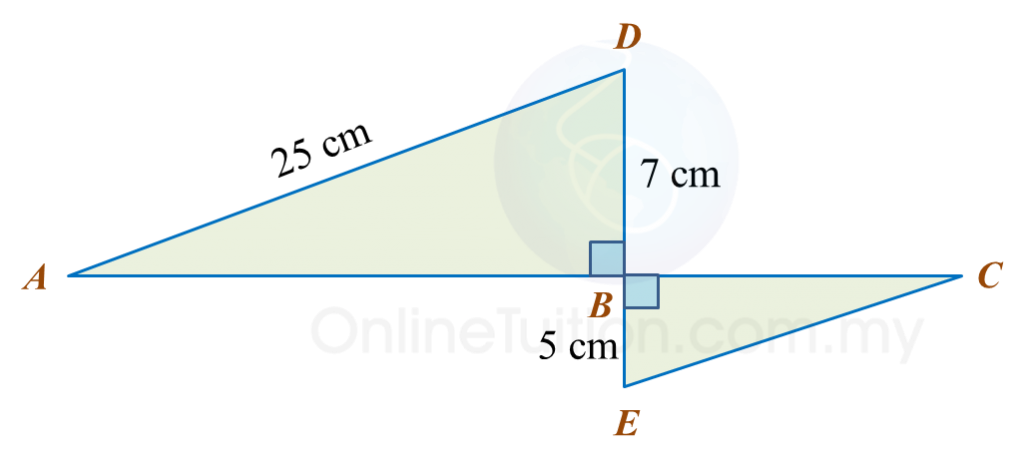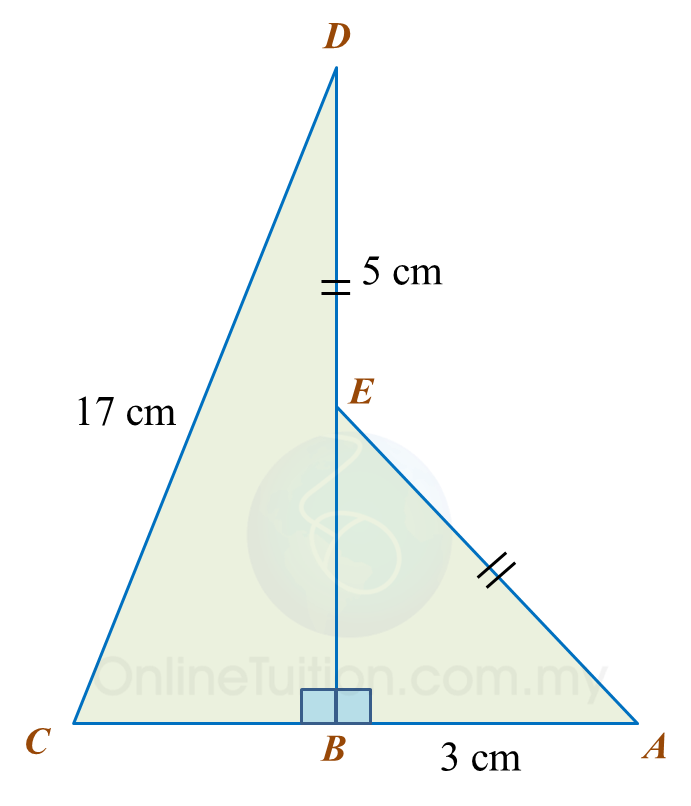6.2.2 Pythagoras’ Theorem, PT3 Focus Practice

Question 6:
In diagram below, ABCH is a square and DEFG is a rectangle. HCDE is a straight line and HC = CD.Find the perimeter, in cm, of the whole diagram.

Solution:

Question 7:
In the diagram, ABC and EFD are right-angled triangles.Calculate the perimeter, in cm, of the shaded region.

Solution:

Question 8:
In the diagram, ABD and BCE are right-angled triangles. ABC and DBE are straight lines. The length of AB is twice the length of BC.Calculate the length, in cm, of CE.

Solution:

Question 9:
Diagram below shows two right-angled triangles, ABE and CBD. BED is a straight line.Find the length, in cm, of BC. Round off the answer to two decimal places.

Solution:

Question 10:
Diagram below shows two right-angled triangles, ABC and ADE. ACD is a straight line.Find the length, in cm, of AE. Round off the answer to one decimal places.

Solution: